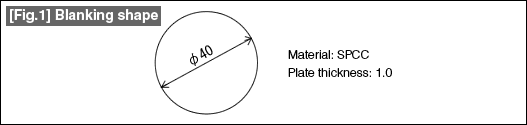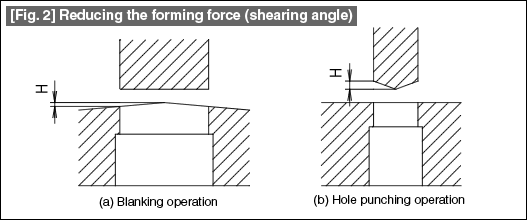# Blanking Force

Thursday, 09 February 2012 08:11 administrator

Knowing the forming force necessary for carrying out a press blanking operation (shearing operation) is indispensable for selecting the press machine and for carrying out the die and punch design.

The blanking force (P) is obtained with the following equation.

 ■Equation 1 P = L * t * S P: Blanking force (kgf) L: Forming circumference (mm) t: Plate thickness (mm) S: Shear resistance (kgf/mm2）

However, when it is difficult to know the shear resistance (S), it is substituted by a value equal to 80% of the tensile strength (Ts) of the material. In this case the equation  will be as follows.

 ■Equation 2 P = K * L * t * Ts P: Blanking force (kgf) K: Coefficient = 0.8 L: Forming circumference (mm) t: Plate thickness (mm) Ts: Tensile strength (kgf/mm2）

Taking the example of Fig. 1, the blanking force will be as follows. The tensile strength of SPCC is taken as 30 kgf/mm2 in this case.

 P = 0.8 * n * 40 * 1 * 30 = 3014.4 (kgf)In order to reduce the blanking force, there is a method of providing a shearing angle as shown in Fig. 2. The shearing angle is provided in the die in the case of blanking operations and in the punch in the case of hole punching operations. Most often the shearing angle is provided so that the dimension H is roughly equal to or more than the plate thickness. By the way, the blanking force can be reduced by about 30% when the dimension H of the shearing angle is made equal to the plate thickness.#### Technology News

| + - | RTL - LTR